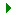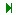Search    VB VB.net C# ASP.net ASP SQL Server VC++ My Links Networking ORACLE MySQL PHP Unix/Linux WinNT/2k/2003 Flash Maya 3D max Photoshop DB2 C/C++/ASM Microcontroller Circuit Design JAVA Wireless/Mobile JScript     Articles from this site only Links only Articles with downloads Articles with screenshot(s)  Code/Page 10 20 30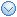User Login Email Password Remember Me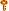Forgot the Password?Services
» Web Development
» Maintenance
» Data Integration/BI
» Information ManagementProgrammingVB (1648)VB.net (736)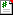C# (15)ASP.net (779)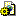ASP (41)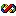VC++ (25)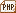PHP (0)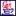JAVA (4)JScript (5)Database
 » SQL Server (708) » ORACLE (5) » MySQL (0) » DB2 (0)Automation
 » C/C++/ASM (11) » Microcontroller (1) » Circuit Design (0)OS/Networking
 » Networking (5) » Unix/Linux (1) » WinNT/2k/2003 (8)Graphics
 » Flash (0) » Maya (0) » 3D max (0) » Photoshop (0)Links
 » ASP.net (2) » PC Interfacing (1) » Networking (4) » SQL Server (4) » VB (23) » VB.net (4) » VC (3) » HTML/CSS/JavaScript (10)Tools
» Regular Expr Tester
» Free Tools

 (Page 14 of 133)3985 Result(s) found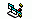Base Conversion module - A module to convert numbers between any bases Total Hit (2983) «Code LangId=1»'----------------------------------------------------------------- ' Module: mBases ' (C) 2000 Trinet Ltd, http://www.trinet.co.uk ' Author: R. Deeming (richard@trinet.co.uk) ' ' Purpose: To provide simple conversion between different ' number ba ....Read More Rating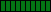Bin - Convert from decimal to binary Total Hit (1791) «Code LangId=1»' convert from decimal to binary ' if you pass the Digits argument, the result is truncated ' to that number of digits ' Function Bin(ByVal value As Long, Optional digits As Long = -1) As String Dim result As String, exponent As Integer ' this is faster than creating t ....Read More RatingBinToDec - Convert from binary to decimal Total Hit (2881) «Code LangId=1»' convert from binary to decimal ' Function BinToDec(value As String) As Long Dim result As Long, i As Integer, exponent As Integer For i = Len(value) To 1 Step -1 Select Case Asc(Mid\$(value, i, 1)) Case 48 ' "0", do nothing Case 4 ....Read More Rating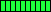BitClear - Clear a bit in a value Total Hit (1668) «Code LangId=1»Function BitClear(ByVal value As Long, ByVal bit As Long) As Long ' simply AND with the negation of the bit mask ' Range checking is performed in Power2() BitClear = (value And Not Power2(bit)) End Function ' Raise 2 to a power ' the exponent must be in the range [ ....Read More RatingBitCount - The number of "1" bits in a number Total Hit (1624) «Code LangId=1» ' The number of 1's in a binary number ' ' This routine is based on the following property ' of binary numbers: n And (n-1) always clears the ' least significant "1" bit in the number Function BitCount (ByVal number As Long) As Integer Do While number number = ....Read More RatingBitSet - Set a bit in a number Total Hit (1801) «Code LangId=1»' Set a bit in a value ' ' NOTE: requires Power2() Function BitSet(ByVal value As Long, ByVal bit As Long) As Long ' simply OR with the bit mask ' Range checking is performed in Power2() BitSet = (value Or Power2(bit)) End Function ' Raise 2 to a power ' the e ....Read More RatingBitTest - Test the value of a bit Total Hit (2340) «Code LangId=1»' Test the value of a bit ' ' NOTE: requires Power2() Function BitTest(ByVal value As Long, ByVal bit As Long) As Boolean ' simply AND with the bit mask ' Range checking is performed in Power2() BitTest = (value And Power2(bit)) End Function ' Raise 2 to a po ....Read More RatingBitToggle - Invert a bit in a value Total Hit (1903) «Code LangId=1»' Toggle a bit in a value ' ' NOTE: requires Power2() Function BitToggle(ByVal value As Long, ByVal bit As Long) As Long ' simply XOR with the negation of the bit mask ' Range checking is performed in Power2() BitToggle = (value Xor Power2(bit)) End Function ....Read More RatingCComplexNumber - A class for dealing with complex numbers Total Hit (1761) «Code LangId=1»Option Explicit '------------------------------------------ ' A class for dealing with complex numbers '------------------------------------------ ' The main properties Public Real As Double Public Imaginary As Double ' Initialize this complex number ' (returns Me) Fu ....Read More RatingCeiling - The integer equal or higher than a given value Total Hit (1708) «Code LangId=1»' Returns the integer equal or higher than its argument Function Ceiling(Number As Double) As Long Ceiling = -Int(-Number) End Function «/Code» RatingCelsiusToFahrenheit, FahrenheitToCelsius - Convert temperature values Total Hit (1804) «Code LangId=1»' Returns the integer equal or higher than its argument Function Ceiling(Number As Double) As Long Ceiling = -Int(-Number) End Function «/Code» RatingCombinations - The number of combinations of N objects in groups of N Total Hit (1565) «Code LangId=1»' number of Combinations of N objects in groups of M ' ' Note: requires the FACTORIAL routine Function Combinations(ByVal Objects As Long, ByVal GroupSize As Long) As Double Combinations = (Factorial(Objects) / Factorial(Objects - GroupSize)) / _ Factorial(GroupSize ....Read More RatingCot, Sec, Csc - Missing trig functions Total Hit (1998) «Code LangId=1»' Cotangent of an angle Function Cot(radians As Double) As Double Cot = 1 / Tan(radians) End Function ' Secant of an angle Function Sec(radians As Double) As Double Sec = 1 / Cos(radians) End Function ' cosecant of an angle Function Csc(radians As Double) As ....Read More RatingCrc16 - Evaluate the 16-bit CRC of an array of bytes Total Hit (2991) «Code LangId=1»Option Explicit ' Evalutate the 16-bit CRC (Cyclic Redundancy Checksum) of an array of bytes ' ' If you omit the second argument, the entire array is considered Function Crc16(cp() As Byte, Optional ByVal Size As Long = -1) As Long Dim i As Long Dim fcs As Long Static ....Read More RatingDec2Any - Convert a decimal number to any other base Total Hit (2101) «Code LangId=1»' convert a number to any base ' BASE can be in the range 2-36 Function Dec2Any(ByVal number As Long, ByVal base As Integer) As String Dim index As Long Dim digits As String Dim digitValue As Long ' check base If base < 2 Or base > 36 Then Err.Raise ....Read More RatingDecToFrac - Converts a decimal number into a fraction Total Hit (1213) «Code LangId=1»' Converts a decimal value into fractional parts as integers ' (based on the concept of Continued Fractions) ' Examples of usage: ' Call DeclToFrac(0.125, a, b) ' 1 and 8 are returned in a & b ' Call DecToFrac(5/40, a, b) ' 1 and 8 are also returned ' Call DecToFrac(2/3 ....Read More RatingDegreesToRadians, RadiansToDegrees - Convert from radians to degrees and back Total Hit (1181) «Code LangId=1»' convert from degrees to radians Function DegreesToRadians(ByVal degrees As Single) As Single DegreesToRadians = degrees / 57.29578 End Function ' convert from radians to degrees Function RadiansToDegrees(ByVal radians As Single) As Single RadiansToDegrees = radia ....Read More Rating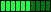Factorial - The factorial of a number Total Hit (1241) «Code LangId=1»' The factorial of a number ' ' if NUMBER is negative or >170 it raises an ' "subscript out of range" error Function Factorial(ByVal number As Long) As Double Static result(170) As Double ' this routine is very fast because it ' caches all the possible resul ....Read More RatingFract - The fractional portion of a number Total Hit (1085) «Code LangId=1»' The fractional part of a floating-point number ' note that negative numbers return negative values Function Fract(number As Variant) As Variant Fract = number - Fix(number) End Function «/Code» RatingGCD - The Greatest Common Divisor of two integers Total Hit (1126) «Code LangId=1»' the Greatest Common Divisor of two integers ' (it uses the Euclide's algorithm) ' if either argument is zero you get a "Division by Zero" error Function GCD(ByVal n1 As Long, ByVal n2 As Long) As Long Dim tmp As Long Do ' swap the items so that n1 >= n2 ....Read More RatingGetPrimeNumbers - Evaluate the first N prime numbers Total Hit (962) «Code LangId=1»' Returns an array with the first N prime numbers ' ' Note: you can easily convert this routine to VB4 and VB5 by ' returning the result array through an argument instead of ' the return value Function GetPrimeNumbers(numberOfPrimes As Long) As Long() Dim found As Long ....Read More RatingHiWord - The most significant word in a Long value Total Hit (1634) «Code LangId=1» Private Declare Sub CopyMemory Lib "kernel32" Alias "RtlMoveMemory" (dest As _ Any, source As Any, ByVal bytes As Long) ' Return the high word of a Long value. Function HiWord(ByVal value As Long) As Integer CopyMemory HiWord, ByVal VarPtr(value) + 2, 2 End Function ....Read More RatingIsPrime - Determine whether a number is prime Total Hit (1120) «Code LangId=1» ' Return True if the number is prime Function IsPrime(ByVal number As Long) As Boolean ' manually test 2 and 3 If number > 3 Then If number Mod 2 = 0 Then Exit Function If number Mod 3 = 0 Then Exit Function End If ' we can now avoid to consi ....Read More RatingLCM - The Least Common Multiple of two integers Total Hit (2651) «Code LangId=1»' the Least Common Multiple of two integers ' (it uses the Euclide's algorithm) ' if either argument is zero you get a "Division by Zero" error ' ' Note: if your app also includes the CGD() function, ' you can simplify the following code as follows: ' LCM = (n1 * n2) ....Read More RatingLog10 - Base-10 logarithm Total Hit (1165) «Code LangId=1» ' Base 10 logarithm Function Log10(number As Double) As Double Log10 = Log(number) / 2.30258509299405 End Function «/Code» RatingLowWord - The least significant word of a Long value. Total Hit (1588) «Code LangId=1»Private Declare Sub CopyMemory Lib "kernel32" Alias "RtlMoveMemory" (dest As _ Any, source As Any, ByVal bytes As Long) ' Return the low word of a Long value Function LowWord(ByVal value As Long) As Integer CopyMemory LowWord, value, 2 End Function «/Code» ....Read More Rating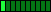MK? And CV? - Convert numbers to strings and back Total Hit (2402) «Code LangId=1» ' This group of routines convert a numeric value into a string ' that contain the internal representation of the number. ' They can be useful when converting outdated QuickBasic programs, ' because that language supported these functions that were ' never ported to Visual Basic ....Read More RatingNormRand - Produce random numbers with normal distribution Total Hit (1300) «Code LangId=1»' VBA's intrinsic Rnd function returns numbers evenly ' distributed between 0 and 1. Each number in that ' interval has equal probability of being returned ' for any given function call. ' This function NormRand returns a random number between ' -infinity and +infinity distib ....Read More RatingPermutations - Number of permutations of N objects in groups of M Total Hit (1129) «Code LangId=1»' number of permutations of N objects in groups of M ' ' Note: requires the FACTORIAL routine Function Permutations(ByVal Objects As Long, ByVal GroupSize As Long) As Double Permutations = Factorial(Objects) / Factorial(Objects - GroupSize) End Function «/Code» ....Read More RatingPower2 - A power of 2 Total Hit (1172) «Code LangId=1» ' Raise 2 to a power ' the exponent must be in the range [0,31] Function Power2(ByVal exponent As Long) As Long Static res(0 To 31) As Long Dim i As Long ' rule out errors If exponent < 0 Or exponent > 31 Then Err.Raise 5 ' initialize the arr ....Read More Rating(Page 14 of 133)3985 Result(s) found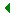1 2 3 4 5 6 7 8 9 10 11 12 13 14 15 16 17 18 19 20 21 22 23 24 25 26 27 28 29 30 ...